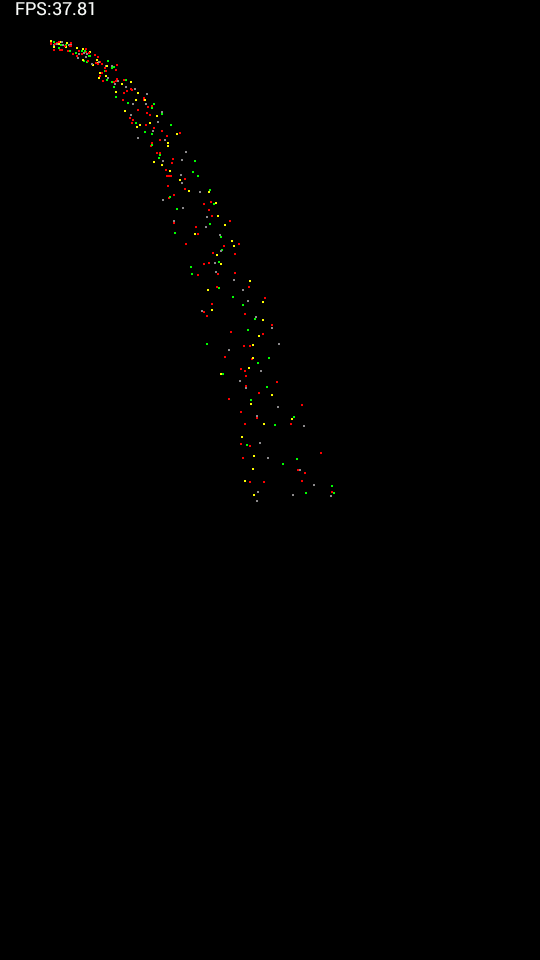瀑布粒子系统/**
* 向粒子集合添加指定个数的粒子对象
*
* @param count
* @param startTime
*/
public void add(int count, double startTime) {
for (int i = 0; i < count; i++) {
int tempColor = this.getColor(i);
int tempR = 1;
// 竖直方向的速度设置为0
double tempv_v =0;
// 随机产生粒子水平方向的速度
double tempv_h = 20 +10 * (Math.random());
int tempX = 50;
// 随机产生粒子Y坐标，90~100之间
int tempY = (int) (50 - 10 * (Math.random()));
// 创建粒子对象
Particle particle = new Particle(tempColor, tempR, tempv_v,
tempv_h, tempX, tempY, startTime);
}